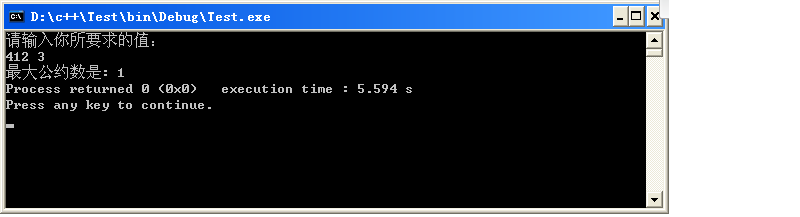• <!... ...if (arguments[i] > max){ max = arguments[i]; } } return max; } var re = getMax(1,3,444,1233); console.log(re);
<!DOCTYPE html>
<html lang="en">

<meta charset="UTF-8">
<meta name="viewport" content="width=device-width, initial-scale=1.0">
<meta http-equiv="X-UA-Compatible" content="ie=edge">
<title>Document</title>
<script>
function getMax(){
var max = arguments;
for(var i = 0;i < arguments.length; i++){
if (arguments[i] > max){
max = arguments[i];
}
}
return max;
}
var re = getMax(1,3,444,1233);
console.log(re);
</script>

<body>

</body>

</html>
展开全文• /* 烟台大学计算机学院 作者：任子仪 ... 问题分析：利用自定义函数求最大公约数 */ #include using namespace std; int gcd(int x,int y) //定义用于两数的最大公约数的函数 { int t,r;
/*
烟台大学计算机学院
作者：任子仪
日期：2013年11月6号
问题描述：自定义函数来求值
样例输入:
样例输出：
问题分析：利用自定义函数求最大公约数
*/
#include <iostream>
using namespace std;
int gcd(int x,int y) //定义用于求两数的最大公约数的函数
{
int t,r;
if(x<y) t=x,x=y,y=t;
while (y!=0)
{
r=x%y;
x=y;
y=r;
}
return x;
}
int main()
{
int a,b,g;
cout<<"请输入你所要求的值："<<endl;
cin>>a>>b;
g=gcd(a,b);
cout<<"最大公约数是: "<<g;

return 0;
}



图片示例：心得体会：自定义函数比前面的难，好好学啊，加油啊。。。。 
展开全文• 假设要在程序中实现求最大值，但是由于求最大值的个数和参数都不确定，因此要针对不同的情况设计不同的方法，这里就用到了函数重载。函数重载函数名相同但是参数的类型和个数不能相同。下面的例子分别是两个数的...
<span style="font-family: Arial, Helvetica, sans-serif; background-color: rgb(255, 255, 255);">假设要在程序中实现求最大值，但是由于求最大值的个数和参数都不确定，因此要针对不同的情况设计不同的方法，这里就用到了函数重载。函数重载函数名相同但是参数的类型和个数不能相同。下面的例子分别是求两个数的最大值，三个数的最大在值数组中的最大值；</span>

public class Text
{
public static void main(String []args)
{
int max1 = max(8,1);
int max2 = max(4,66,1);
int arr[] = {45,65,1 ,23,74,11,0,12};
int max3 = max(arr);

System.out.println("max1 = "+max1);
System.out.println("max2 = "+max2);
System.out.println("max3 = "+max3);
}
public static int max(int x, int y)
{
return x>y?x:y;
}
public static int max(int x, int y,int z)
{
return max(x,y)>z?max(x,y):z;
}
public static int max(int arr[])
{
int max=arr;
for(int i=0; i<arr.length; i++)
{
max=max(max,arr[i]);
}
return max;
}<pre name="code" class="html">if(x>y)
{
return x;
}
else
{
return y;
}
}

以上的里也用到了三目运算符即 x>y?x:y;它相当于
<pre name="code" class="html">if(x>y)
{
return x;
}
else
{
return y;
}由于博主对函数重载目前不是很精通，以后会详细介绍


展开全文java
• 今天学习了遗传算法，其最大的特点就是能取全局最优值，但是算法随机性高，对连续定义域很难求得精确解，本文仅参考遗传算法的思想，在整数范围内，来函数全局最大值 首先回顾一下算法流程 (b)方法在(a)的...


遗传算法的简单实现
今天学习了遗传算法，其最大的特点就是能求取全局最优值，但是算法随机性高，对连续定义域很难求得精确解，本文仅参考遗传算法的思想，在整数范围内，来求取函数全局最大值

首先回顾一下算法流程(b)方法在(a)的基础上，选择保留一部分父代个体，防止优秀基因的丢失，我在这里使用的是经典遗传算法，在下面这种简单的二次函数方面效果不错
clc;
clear;
%设置起始计时器
tic;
%定义群体个数num（设为偶数），定义自变量数n，定义自变量取值范围[1,top],
num=160;n=4;top=12;
%定义用来储存目标结果的向量
F_result=zeros(1,num);
%定义种群最大值，平均值
F_max=[];F_mean=[];F_min=[];
%随机生成num个个体，即生成初始种群
for i=1:num
for j=1:n
X{i}(j)=unidrnd(top);
end
F_result(i)=f(X{i});     %请先自定义f函数
end
i=1;
%选择使函数f较大的前一半个体随机组合，随机交换基因，产生下一代，直到群体数复原
while(1)
%取群体最大值，平均值，最小值作为观察对象
F_max(i)=max(F_result);F_mean(i)=mean(F_result);F_min(i)=min(F_result);
if (F_max(i)==F_mean(i))
break;
end
%选择前一半表现优秀的个体杂交产生下一代
F_tem=F_result;   %临时存储F的结果用于排序
[result,coo]=sort(F_tem);
t=unidrnd(num/2); %基因交换循环过程中发生变异的某时刻
for j=1:num/2    %基因交换循环过程，子代将完全取代老一代
a=unidrnd(num/2)+num/2;    %随机选择两个表现优秀的不同个体
b=unidrnd(num/2)+num/2;
if(a==b)
j=j-1;
continue;
end
%选择与重组
X{coo(j)}=[X{coo(a)}(1) X{coo(a)}(2) X{coo(b)}(3) X{coo(b)}(4)];
F_result(coo(j))=f(X{coo(j)});
X{coo(j+num/2)}=[X{coo(b)}(1) X{coo(b)}(2) X{coo(a)}(3) X{coo(a)}(4)];
F_result(coo(j+num/2))=f(X{coo(j+num/2)});
%变异
if(j==t)
c=unidrnd(n);    %任选一位变异
d=unidrnd(top);  %任变异成定义域内某值
X{coo(j)}(c)=d;
F_result(coo(j))=f(X{coo(j)});
X{coo(j+num/2)}(c)=d;
F_result(coo(j+num/2))=f(X{coo(j+num/2)});
end
end
i=i+1;
end
plot(F_max,'r');
hold on;
plot(F_mean,'g');
hold on;
plot(F_min,'b');
toc;


下面是运行结果展开全文算法
• 文章目录遗传算法求最大值主程序相关函数初始化种群逆转录（2进制到目标函数值）逆转录基因逆转录染色体计算基因型和表现型（10进制函数自变量及函数值）计算适应度种群变化复制交叉变异种群最适个体 遗传算法...matlab 遗传算法
• 编写函数求一个数组中数组元素的最大值，要求必须用递归方法解决。 输入格式: 数组中的数字均为整型数，输入的第一个数为数组长度，后续为数组的所有元素。 输出格式： 该数组的最大值 输入样例： 5 92 18 77...
• import java.util.Scanner; public class Main{ public static int find(int a[]) {//定义三个方法，重载三次 ... if(max&amp;amp;lt;a[i]) { max=a[i]; } } return max; } pu...
• 利用python进行求解，求解的要求是不能使用python内部封装好的函数例如：max way1: def findmax(data,n): if n==1: return data else: maxi=data for i in data[1:]: if maxi&lt;i: ...max
• ## Matlab遗传算法求函数最大值

万次阅读 热门讨论 2016-10-13 20:06:45
主函数函数main.mglobal Bitlength%定义3个全局变量 global boundsbegin global boundsend boundsbegin=-2; boundsend=2; precision=0.0001;%运算精确度 Bitlength=ceil(log2((boundsend-boundsbegin)'./precision))...matlab 算法 遗传算法 数学建模
• 下面讲述如何利用遗传算法解决一个二元函数最大值求解问题。 问题 二元函数如下： # 画出图像如下 from mpl_toolkits.mplot3d import Axes3D import numpy as np from matplotlib import pyplot as plt fig...
• 用遗传算法求函数f(a,b)=2a x sin(8PI x b) + b x cos(13PI x a)最大值，a:[-3,7]，b:[-4:10] 实现步骤： 初始化种群 计算种群中每个个体的适应值 淘汰部分个体（这里是求最大值，f值存在正值，所以淘汰所有负值） ...
• 方法一： #include int max(int a,int b); int main() { int a,b; printf("请输入a,b:"); scanf("%d%d",&a,&b); printf("max=%d\n",max(a,b));... if(a>b) c=a; else c=b; return c; }
• 思路：定义两个函数，分别用于出四个数字和两个数字中的最大值。 程序代码： #include <stdio.h> #include <windows.h> int max4(int i,int j,int k,int l) { int max; max=max2(i,j); max=max2...
• 在Excel中有sumif,countif等函数可以实现特定条件下数值的加总和计数，那么如何在一个或多个条件下出此时的最大值或者最小值呢？ 其实sumif函数和countif函数实际上都是可以由sum/count+if函数通过数组实现的，...excel
• 一元多值函数求极值在实际科研中具有很重要的作用，其中求最大值最小值又有相似的地方，只要可以出两者中的一者，对函数加一个负号就可以出另一个最值。 下面是一个利用fminbnd函数，结合图形观察得出...数值计算 matlab
• <script> function getMax() { var max = arguments; for (var i = 1; i < arguments.length; i++) { if (arguments[i] > max) { max = arguments[i]; } }javascript
• ## 【数组】求最大值及其下标

万次阅读 多人点赞 2019-03-23 10:48:30
题目：求最大值及其下标。 要求：找出给定的n个数中的最大值及其对应的最小下标(下标从0开始)。 输入格式： 　输入在第一行中给出一个正整数n（1<n≤10）。第二行输入n个整数，用空格分开。 输出格式： 　在...数组
• #include&lt;iostream&gt;#define N 20using namespace std;void Max(int a[],int n){ int i,max; max=a; for(i=1;i&...n个整数中最大值为"&lt;&lt;max&lt;&...
• 分治法求最大值与次大值程序要求函数设计完整代码运行示例 程序要求 采用分治法含n个实数的序列中的最大元素和次大元素 函数设计 1、 void Comp(double arr[],int low,int high,double &max1,double &max2...算法设计与分析 分治法
•   本次分享将讲述如何利用Python中的reduce函数对序列最值以及排序。   我们用reduce函数对序列最值的想法建立在冒泡排序的算法上。先上例子？ from functools import reduce from random import randint ...Python
• java利用数组三个数中的最大值 思想 1.定义数组 2.写get方法 3.函数返回最大值，输出最大值 public class getMax { public static void main(String[] args) { int[] num = {11 , 4 , 65}; int max = getMaxNum...
• if(x>y) { z=x; } else z=y; return z; /*或者 z=x>y?x:z; */ } int main(void) { int max(int x,int y); int a,b,c; printf("please input numbers:\n"); scanf("%d%d",&a,&b); c=max(a,b);//max...c语言
• python接收多个数字，返回最大值自定义函数， 多谢@Gavin_Hall 为我解答我的疑问 max_num = 0 def my_max(x,y): #简单比较两个数大小 global max_num #申请全局变量max_num if x > y: max_num = x else: ...python
• //用函数模板一维数组中的最大和最小值。 #include &lt;iostream&gt; #include "string.h" using namespace std; template &lt;class T&gt; void maxmin(T* x, int n, T* max, T* ...
• /*编写一两个数的最大值函数Max， 要求用模板实现对任意数据类型数据都可应用该函数求取结果， 在main（）函数中分别用整型、实型、字符型数据进行测试。 */ #include&lt;iostream&gt; #include&...
•  本例中，目标函数总取非负值，并且是以求函数最大值为优化目标，故可直接  利用目标函数值作为个体的适应度。  (4) 选择运算  选择运算(或称为复制运算)把当前群体中适应度较高的个体按某种规则或模型...
• 2019独角兽企业重金招聘Python工程师标准>>> ... if(maxvalue < b[i])  maxvalue = b[i];  }  return maxvalue; } 转载于:https://my.oschina.net/u/241930/blog/402697
• 利用遗传算法求函数f(x)=x+10sin(5x)+7cos(4x)f(x) =x+10sin(5x)+7cos(4x)的最大值算法
• # 求最大值 def large(*num): # 定义一个large函数函数的参数为可变参数 ma = num # 初始化最大值 for n in num: if ma < n: # 对参数进行两两比较 ma = n # 对最大值重新赋值 return ma # 返回......